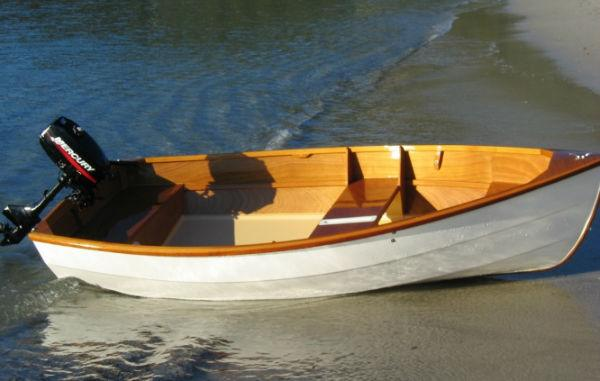# River Crossing Puzzle: Two Ladies and Two Girls

Use your logical skills to solve this riddle.

At Two ladies and two girls need to cross a river in a boat.

The boat is big enough for 1 LADY or 2 girls.

How do they do it?So were you able to solve the riddle? Leave your answers in the comment section below.

Solution to the River Crossing Puzzle:

To simply let us denote the entities involved as follows;

Let,

Girls be called as G
Boat be called as B

Let W be the west coast where everyone is currently present and
E be the east coast where everyone should be at the end.

Step 1:
Let the 2 girls cross from W to E
W [L,L]
E[G,G,B]

Step 2:
Let G return with the boat
W [L,L,G,B]
E[G]

Step 3:
Let L go with B
W [L,G]
E[L,G,B]

Step 4:
Let G return with the boat
W [L,G,G,B]
E[L]

Step 5:
Let the 2 girls cross from W to E
W [L]
E[L,G,G,B]

Step 6:
Let G return with the boat
W [L,G,B]
E[L,G]

Step7:
Let L go with B
W [G]
E[L,L,G,B]

Step 8:
Let G return with the boat
W [G,G,B]
E[L,L]

Step 9:
Let the two girls go in the boat to E
W [empty]
E[L,L,G,G,B]